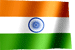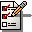RAMESH KRISHNA REDDYOnline Tutorials | PREV | TOP | NEXT

DRONA SERIES
JCL STUDY MATERIAL

CHAPTER - 10

 ``` Control Job Processing (IF THEN ELSE) in JCL There are two ways to control job processing in JCL, One way is by coding COND parameter either on JOB or on EXEC, Second way is , using IF condition. In previous chapters, we have discussed about COND parameter on both JOB as well as EXEC. Now let us discuss about IF condition in JCL Using IF construct is more powerful than COND. - The COND parameter on the first step of a job is ignored However,the IF construct is tested - We can code symbolic parameters in the IF conditions - We can code many types of relational-expressions in IF condition Syntax of IF //name IF < condition > THEN . . //name1 ELSE . . // ENDIF The condition (ralational expression) consists of: Comparison operators Logical operators Not (�) operators Relational expression keywords. Example JCL //EXAMPLE JOB //S1 EXEC MYPROC1 //COND01 IF RC = 0 THEN //C01OK EXEC MYPROC2 //CONDE ELSE //C01ELS EXEC MYPROC3 // ENDIF //* copyright www.mainframegurukul.com EXPLANATION In above example, S1 is first job step In 3 rd line, we check the Recturn code of S1 step if return code is zero then C01OK step will execute else step C01ELS will going to execute A Keyword List  Keyword  Purpose  ABEND  Tests for an abnormal end of a program  �ABEND  Tests that an abnormal end of a program did not occur  ABENDCC  Examines an ABEND condition code  RC  Examines a return code  RUN  Tests if a job step executed  �RUN  Tests if a job step did not execute All the keywords can include a stepname and procstepname to refine the test to a specific job step. The format is stepname.procstepname.keyword. RC - checks a return code. EXAMPLE JCLs IF RC = 0 THEN IF STEP1.RC < 12 THEN If you not given the stepname, then the highest return code from all job steps is taken for checking. ABENDCC - Checks an ABEND condition code Using ABENDCC we can check System/User completion codes EXAMPLE IF ABENDCC = S0C7 THEN Suppose you want to check error code of particular step, give stepnname.ABENDCC, If you not given the stepname, most recent ABEND code that occured is taken for checking ABEND - checks for an abnormal end of a program EXAMPLE JCL IF ABEND THEN IF STEP4.PROCAS01.ABEND = TRUE THEN If you not given any stepname, all steps prior to this condition will be checked RUN - to check whether a job step executed or not EXAMPLE JCL Suppose there is a job which contains STEP2, STEP3,STEP4 I have an if condition for STEP2 and STEP3, if condition is true then STEP2 will be executed, If condition is false then STEP3 will be executed I want to execute STEP4 if STEP2 executes, we can code in the following way //CHE01 IF STEP2.RUN THEN //STEP4 EXEC MYPROC1 // ENDIF //** copyright www.mainframegurukul.comEither the THEN clause or ELSE clause must contain at least one EXEC statement.You can nest another IF construct after the THEN clause or the ELSE clause. You can nest IF/THEN/ELSE/ENDIF statement constructs up to 15 levels of nesting. NEXT CHAPTER TOPIC : Restarting and checkpoint in jcl ```

Visit jcl books section in this site for good books